Integral curve, flow

We define a specific vector field U. The differential equations for the integral curve of U are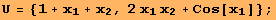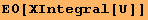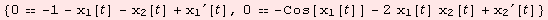Both of the following lines do the same, namely solve an integral curve α:I→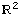numerically. The first curve starts at the initial point α(0)={1,0}. The curve is parametrized on the interval I={-1,1}.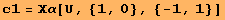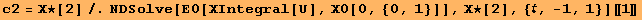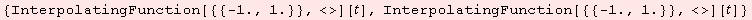We plot the vector field and the integral curves.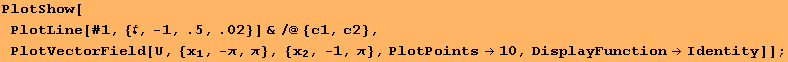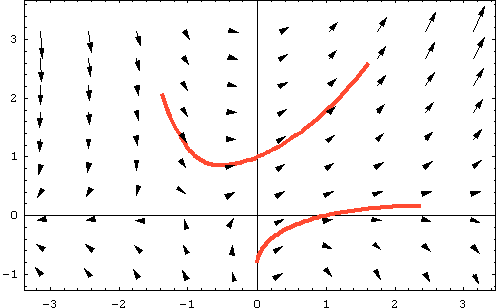The unit circle gets transported by the flow of U below.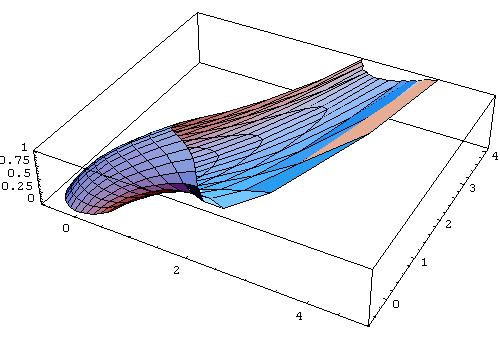We compute the differential of the flow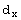ψ(t,x)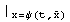of a vector field V along the integral curve with initial point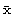∈M. The differential depends on the parameter t∈I. Below, we chose={1,1} and I={-1,1}.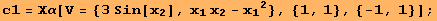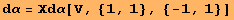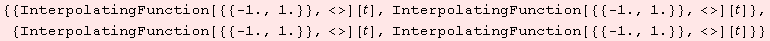Here comes the picture.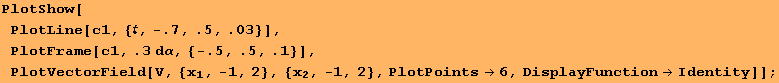Created by Mathematica  (December 22, 2006)